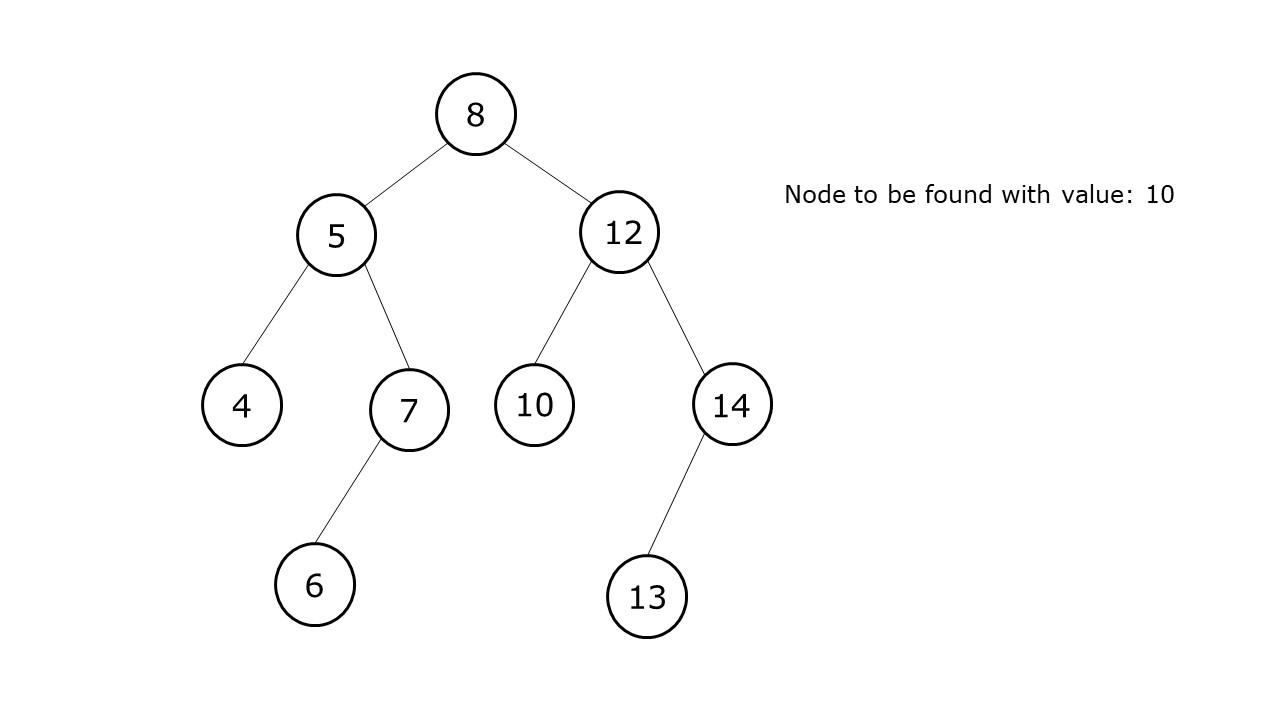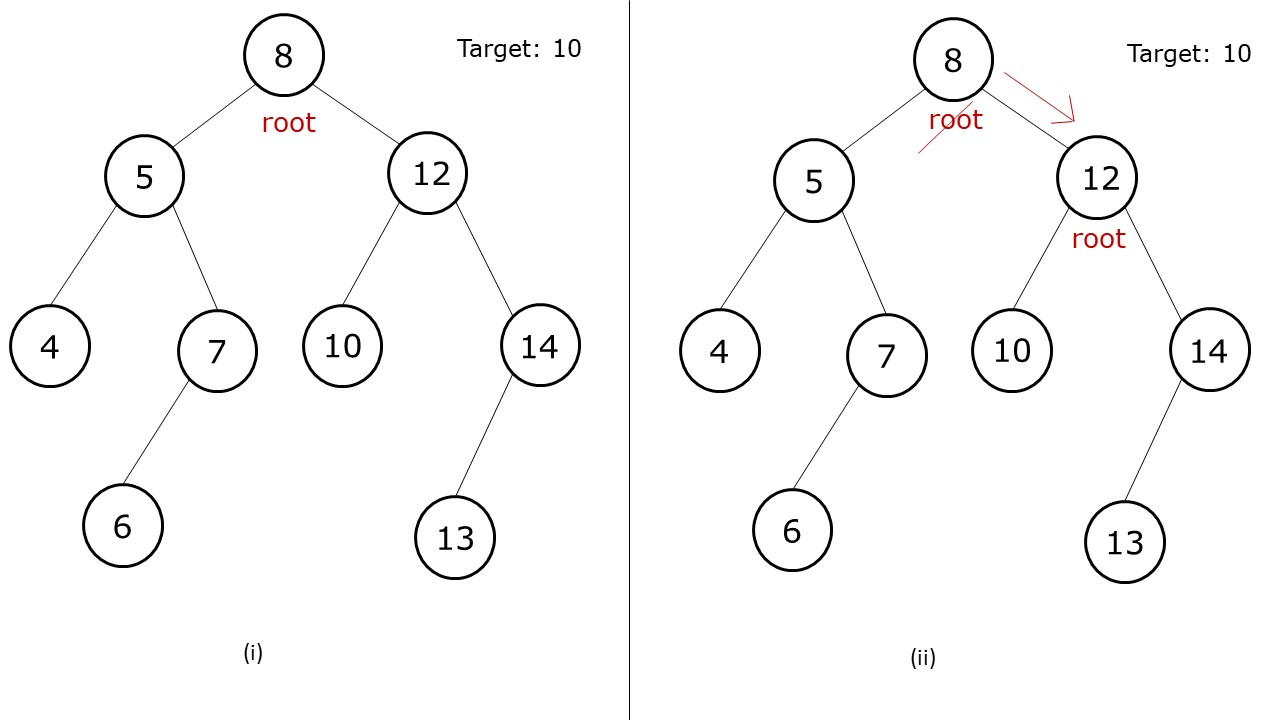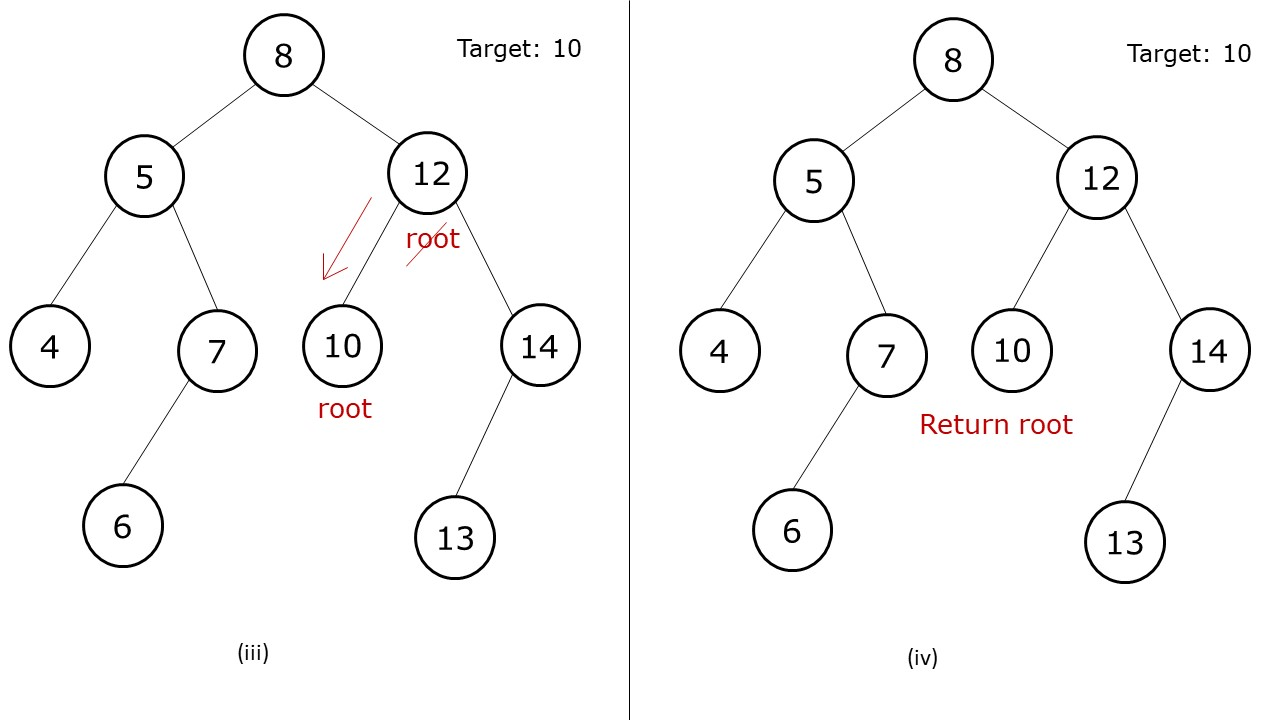# Search in a Binary Search Tree

Write a program to search a node with a given value in a binary search tree.

```Example:```

Disclaimer: Don’t jump directly to the solution, try it out yourself first.

## Intuition:

We are given a binary search tree. In a binary search tree, for every node the following property is satisfied:

Values in the left subtree < Value of node < Values in the right subtree

Therefore, whenever we are at a node, if its value is equal to the value we are searching for,(say target),  we have found our answer and we can return the node address. Else, we can compare the target value to the node’s value. If the target value is less than it we will find our answer in the left subtree else we will find it in the right subtree. At every step, we decrease our search space by half, which is nothing else than binary search.

## Approach:

The algorithm steps can be stated as:

• Set a while loop which runs till the time root is not NULL and root’s value is not equal to the target value we are searching for.
• Inside the while loop, if the target value is less than the root’s value, move root to its left child, else move root to its right child.
• When the while loop ends, return root as the answer.

Dry Run:

# When the target value is present inside the tree(i) First we set the while loop to run.

(ii) As the target is greater than the root’s value(8), we assign the root its right child.(iii) As the target’s value is lesser than the root’s value(12), we assign the root to its right child.

(iv) As the target’s value is equal to the current root’s vale, we break from the while loop and return this root’s address as our answer.

Code:

## C++ Code

``````#include <bits/stdc++.h>

using namespace std;

struct node {
int data;
struct node * left, * right;
};

struct node * newNode(int data) {
struct node * node = (struct node * ) malloc(sizeof(struct node));
node -> data = data;
node -> left = NULL;
node -> right = NULL;

return (node);
}

node* searchBST(node* root, int target) {
while(root != NULL && root->data != target){
root = target<root->data? root->left:root->right;
}
return root;
}

int main() {

struct node * root = newNode(8);
root -> left = newNode(5);
root -> left -> left = newNode(4);
root -> left -> right = newNode(7);
root -> left -> right -> left = newNode(6);
root -> right = newNode(12);
root -> right -> left = newNode(10);
root -> right -> right = newNode(14);
root -> right -> right ->left = newNode(13);

node* found = searchBST(root,10);

if(found != NULL)
cout<<"Node value with given target found";
else cout<<"Node value with given target not found";

return 0;
}
``````

Output:

Node value with given target found

Time Complexity: O(logN)

Reason: The time required will be proportional to the height of the tree, if the tree is balanced, then the height of the tree is logN.

Space Complexity: O(1)

Reason: We are not using any extra space.

## Java Code

``````import java.util.*;

class Node {
int data;
Node  left, right;
Node(int data)
{
this.data=data;
left=null;
right=null;
}
}

class TUF{
static Node searchBST(Node root, int target) {
while(root != null && root.data != target){
root = target<root.data? root.left:root.right;
}
return root;
}

public static void main(String args[]) {

Node root = new Node(8);
root . left = new Node(5);
root . left . left = new Node(4);
root . left . right = new Node(7);
root . left . right . left = new Node(6);
root . right = new Node(12);
root . right . left = new Node(10);
root . right . right = new Node(14);
root . right . right . left = new Node(13);

Node found = searchBST(root,10);

if(found != null)
System.out.println("Node value with given target found");
else System.out.println("Node value with given target not found");

}
}
``````

Output:

Node value with given target found

Time Complexity: O(logN)

Reason: The time required will be proportional to the height of the tree, if the tree is balanced, then the height of the tree is logN.

Space Complexity: O(1)

Reason: We are not using any extra space.

Special thanks to Anshuman Sharma for contributing to this article on takeUforward. If you also wish to share your knowledge with the takeUforward fam, please check out this article